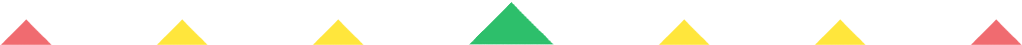Player 1
vs
Player 2
0:00
Opponent history
x
xCorrect
0
Wrong
0
Correct!
Wrong!
Help Sheet for

• 0 + 7 = 7
• 1 + 7 = 8
• 2 + 7 = 9
• 3 + 7 = 10
• 4 + 7 = 11
• 5 + 7 = 12
• 6 + 7 = 13
• 7 + 7 = 14